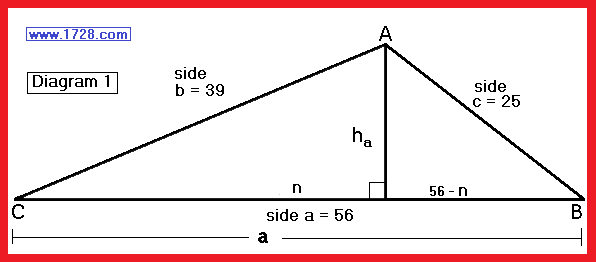Triangle   Height   Calculator Click here to see how triangle heights are calculated. All triangles have 3 altitudes and this calculator will determine all three. Input side a Input side b Input side c Click "RESET" to clear all the boxes.  Perimeter= Semi-Perim=      Area=   Height a=  Height b=  Height c=  Angle A=  Angle B=  Angle C=Significant Figures >>> The default numeric display is 5 significant figures. Numbers > -1,000 and <1,000 are NOT in scientific notation. You can change the display option by changing the number in the box above. Entering a zero eliminates all formatting. Return To Home Page Copyright © 1999 -     1728 Software Systems